#### 期刊菜单

Application of Fuzzy Adaptive Internal Model PI Controller in Control System of Small Biomass Boiler
DOI: 10.12677/DSC.2023.122011, PDF , HTML, XML, 下载: 102  浏览: 176  科研立项经费支持

Abstract: Biomass boiler is the main heat energy conversion equipment, and the boiler temperature is one of the important indexes to evaluate the operation status of the equipment, and the quality of its control system directly affects the safety and economy of the boiler. Considering the problems of nonlinearity, large time delay, strong coupling and multi-interference in the temperature control system of biomass fuel boiler, a temperature control strategy based on fuzzy adaptive internal model PI control is proposed. The algorithm adopts fuzzy adaptive PID control, combined with internal model control algorithm and low-order filter, and carries out systematic simulation experiments through MATLAB toolbox. The experimental results show that compared with conventional PI, fuzzy PI and internal model PI control methods, the controller can greatly improve the control quality of the temperature control system, with fast response, accurate control and strong robustness, and can be applied to industrial production.

1. 引言

2. 锅炉温度系统的数学模型

$G\left(S\right)=\frac{K{\text{e}}^{-\tau S}}{TS+1}$ (1)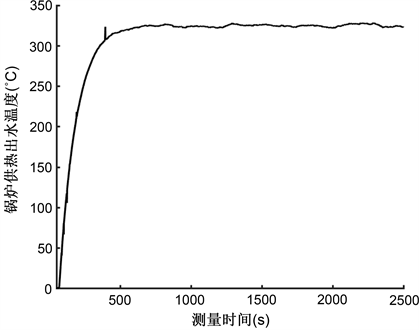Figure 1. Temperature skyrocketing curve

$K=\frac{y\left(\infty \right)}{1\left(\infty \right)}$ (2)

${T}_{ar}=\frac{{\int }_{0}^{\infty }\left(y\left(\infty \right)-y\left(t\right)\right)\text{d}t}{K}=T+\tau$ (3)

$T=\frac{e{\int }_{0}^{{T}_{ar}}y\left(t\right)\text{d}t}{K}$ (4)

$\tau ={T}_{ar}-T$ (5)

$1\left(\infty \right)$ ——系统稳定时的输入信号；

${T}_{ar}$ ——平均滞留时间。

$G\left(s\right)=\frac{5{\text{e}}^{-69s}}{117s+1}$ (6)

3. 模糊自适应内模PID设计

3.1. 常规PID控制

PID控制是一种运用比例、积分、微分对系统偏差进行处理的线性组合控制器。生物质锅炉温度控制器的任务就是保证出水温度稳定在预设值，且能够将控制过程中出现的各种干扰迅速消除，因而对控制品质要求较高，不允许被调量出现静差  。故选用PI控制，其算法表达式如下

$u\left(t\right)={K}_{p}\left(e\left(t\right)+\frac{1}{{T}_{i}}{\int }_{0}^{t}e\left(t\right)\text{d}t\right)$ (7)

$C\left(s\right)=\frac{U\left(s\right)}{E\left(s\right)}={K}_{p}\left(1+\frac{1}{{T}_{i}s}\right)$ (8)

PI控制结构如图2所示，PI控制参数好坏直接影响到生物质成型燃料锅炉温度控制系统的控制品质，因此选用合适的控制参数至关重要。Figure 2. Conventional PI control structure

3.2. 内模PI控制

3.2.1. 内模控制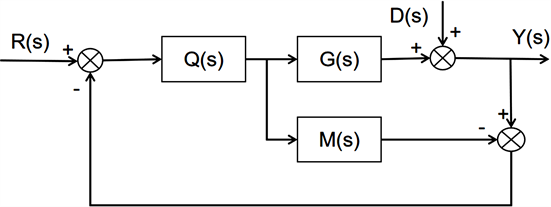Figure 3. Internal mold control structure

$Q\left(s\right)={M}_{-}^{-1}\left(s\right)f\left(s\right)$ (9)

3.2.2. 一阶惯性加纯滞后系统的内模PI控制设计

$G\left(s\right)=\frac{K{\text{e}}^{-\tau s}}{Ts+1}$ (10)

$Q\left(s\right)=\frac{Ts+1}{k{\left({T}_{f}s+1\right)}^{2}}$ (11)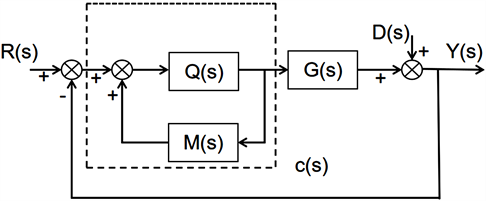Figure 4. Internal model control system equivalent model

$C\left(s\right)=\frac{Q\left(s\right)}{1-Q\left(s\right)M\left(s\right)}$ (12)

$C\left(s\right)={K}_{p}\left(1+\frac{1}{{T}_{i}s}\right)\frac{1}{\epsilon s+1}$ (13)

${K}_{p}=\frac{T}{K\left(2{T}_{f}+\tau \right)}\text{,}{T}_{i}=T\text{,}\epsilon =\frac{{T}_{f}^{2}}{2{T}_{f}+\tau }$ (14)

3.3. 模糊自适应内模PI控制器设计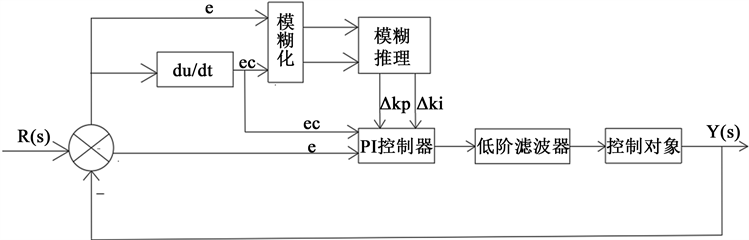Figure 5. Fuzzy adaptive internal model PI control structure

3.3.1. 隶属度函数建立

3.3.2. 模糊控制器规则和决策方法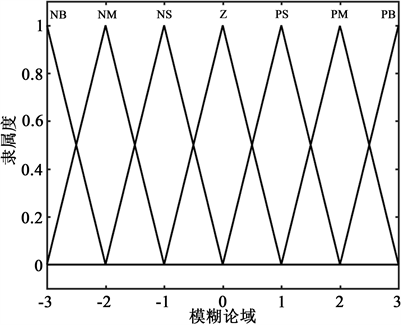Figure 6. Input and output membership function curvesTable 1. Fuzzy control rules for ΔKpTable 2. Fuzzy control rules for ΔKi

${{K}^{\prime }}_{p}={K}_{p}+\Delta {K}_{p}$ (15)

${{K}^{\prime }}_{i}={K}_{i}+\Delta {K}_{i}$ (16)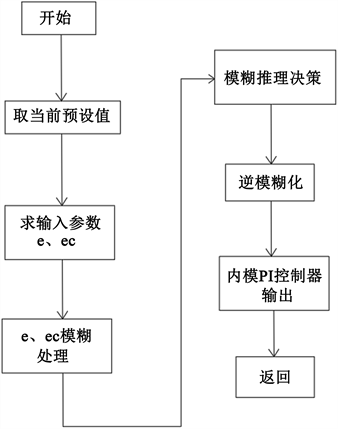Figure 7. The progress of Fuzzy adaptive internal model PI controller

4. 模糊自适应内模PI控制仿真

4.1. 仿真实验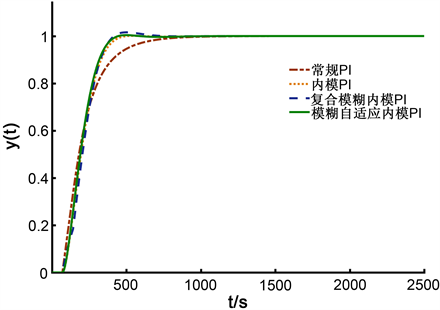Figure 8. System output response curves under normalTable 3. Performance ISTE indication of different control methods under normal

4.2. 扰动实验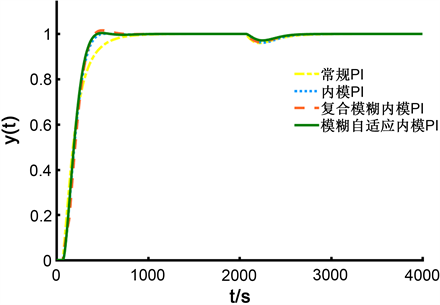Figure 9. System output response curves under disturbance

5. 结论

《基于模型预测控制的生物质取暖炉排放智能控制系统研究》河南省国际科技合作项目(项目编号：232102521011)。

NOTES

*通讯作者。

  解云翔. 中国生物质能发展现状及应用探究[J]. 化学研究, 2022, 33(6): 555-560. https://doi.org/10.14002/j.hxya.2022.06.013  闫景凤. 生物质锅炉应用现状分析[J]. 农机使用与修, 2023(1): 56-58. https://doi.org/10.14031/j.cnki.njwx.2023.01.015  叶政. PID控制器参数整定方法研究及其应用[D]: [硕士学位论文]. 北京: 北京邮电大学, 2016.  花成钰. 生物质锅炉控制系统设计[D]: [硕士学位论文]. 哈尔滨: 哈尔滨工业大学, 2019. https://doi.org/10.27061/d.cnki.ghgdu.2019.005415  潘祥亮, 罗利文. 模糊PID控制在工业锅炉控制系统中的应用[J]. 微计算机信息, 2004(7): 1-2.  孙君曼, 谢泽会, 李莉萍, 冯广军. 基于生物质锅炉的模糊自适应PID控制系统仿真研究[J]. 锅炉技术, 2010, 41(1): 9-12+15.  尚继良, 王晓燕, 于玮. 内模控制在锅炉燃烧系统中的应用研究[J]. 微计算机信息, 2007(34): 41-42+62.  郑元坤. 生物质成型燃料锅炉燃烧控制系统研究[D]: [硕士学位论文]. 淄博: 山东理工大学, 2020. https://doi.org/10.27276/d.cnki.gsdgc.2020.000233  纪志成, 沈艳霞, 姜建国. 一种新型的无刷直流电机调速系统的模糊PI智能控制[J]. 电机与控制学报, 2003(3): 248-254.  赵辉. 基于内模控制原理的PID控制器设计[D]: [硕士学位论文]. 天津: 天津大学, 2005.  王述彦, 师宇, 冯忠绪. 基于模糊PID控制器的控制方法研究[J]. 机械科学与技术, 2011, 30(1): 166-172. https://doi.org/10.13433/j.cnki.1003-8728.2011.01.035  邢胜林, 瞿佳琪. 基于模糊内模控制的锅炉汽包水位控制仿真研究[J]. 电工技术, 2022(18): 1-3.  郭奇, 任芳, 杨田锋, 等. 模糊自适应内模控制在电加热锅炉温度控制中的应用研究[J]. 热能动力工程, 2011, 26(4): 449-452+496.  叶文通. 模型参考模糊自适应内模控制及其在电厂主汽温控制中的应用研究[D]: [硕士学位论文]. 杭州: 浙江工业大学, 2009.  郭瑞君, 张国斌, 纪煜, 等. 基于模糊自适应内模控制的主蒸汽温度控制系统研究[J]. 中国电力, 2018, 51(12): 118-123.